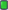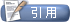MATLAB中国论坛|MATLAB爱好者之家—不仅仅是MATLAB![求助] 用For函数求解方程的泰勒展开，然后求其在x=1处的值。但不知哪错了
 用户名 记住信息 密码
 注册账号 论坛帮助 会员列表 日历事件 搜索 今日新帖 标记版面已读

 Mathematica论坛 Mathematica是比较流行的符号运算软件之一

 LinkBack 主题工具 显示模式2015-10-13, 03:27 AM #1 初级会员   注册日期: 2015-10-11 帖子: 1 感谢他人: 0 有 0 帖获得 0 感谢 声望力: 0用For函数求解方程的泰勒展开，然后求其在x=1处的值。但不知哪错了 问题：牛顿迭代法求方程x-cos(x)=0的泰勒展开（展开20次），然后求其在x=3附近的值，进而给其赋值x=1，求其f（x=1）处的值，但不知哪错了。 跪求指正！ 解答： Clear[x0]; Clear[i]; x0 = 3; f[x_] = x - cos (x); g[x_] = 0; S[x_] = f[x0]; For[i = 1, i <= 20, i++, g[x_] = D[f[x], i]; S[x] = S[x] + g[x0]*(x - x0)^i/i!] S 但是求出来不对。主题工具显示可打印版本邮寄本页给好友 显示模式平板模式切换到混合模式切换到树形模式发帖规则 您不可以发表新主题 您可以发表回复 您不可以上传附件 您不可以编辑自己的帖子 启用 BB 代码 论坛启用 表情符号 论坛启用 [IMG] 代码 论坛禁用 HTML 代码Trackbacks are 禁用 Pingbacks are 禁用 Refbacks are 启用 版面规则

 -- 简体中文 -- 繁體中文 -- English (US) 联系我们 - MATLAB中国论坛|MATLAB爱好者之家 - 论坛存档 - 返回顶端

Powered by vBulletinSEO by vBSEO ©2009, Crawlability, Inc.